# Locking in a quadrilateral element

Home » Knowledge Base » General » Elements » Locking in a quadrilateral element

Locking in linear quadrilateral elements occurs when they are subjected to bending. For simplicity, letês examine the simplest case of a quad in bending.

The element is rectangular with a height 2h and a length 2b. In the x-y plane, the origin is located at the centroid of the element (see Figure 5).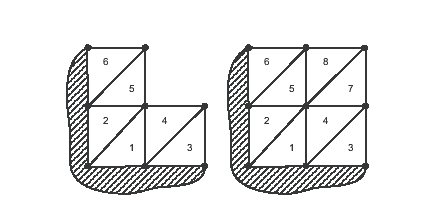Figure 3: A sequence of triangular meshes that are completely locked.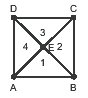Figure 4: Crossed triangles donet lock like the previous conìgurations.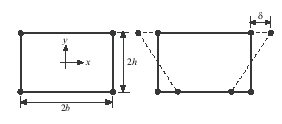Figure 5: The geometry and kinematics of a quadrilateral element in a pure bending node.

The neutral axis is the line y = 0, and cross-sections, which are initially perpendicular to the neutral axis, are deìned by lines of constant x. If the element is subjected to applied moments on the left and right edges, the resulting deformation is bilinear. As shown, it is symmetric about x = 0 and may be characterized by , the displacement of node 3 in the x direction.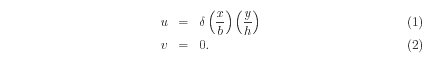Differentiating, the strain, as a function of x and y, is## Shear Locking

Beam theory says that as the thickness of a beam approaches zero, the shear strain goes to zero, and all the load is carried by ε 11. However, the ratio of the shear strain to the tensile strain in the quadrilateral element is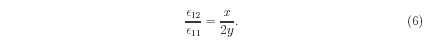The integration locations for 2 x 2 Gauss quadrature are (±h sqrt(3),±b sqrt(3)) in the physical coordinate system. At the integration points, the ratio of thestrains will therefore be proportional to b/h. Clearly, as h/b approaches zero, the ratio 12/ 11 approaches inìnity, and the element locks up because the shear strain, and not the tensile strain, is carrying the load. For a pure bending mode, the shear strain is zero along the line x = 0 according to Equation 5, and along the same line, ε 11 is linear in y, which are the theoretical strains. Two-node beams which are formulated by degenerating solid elements (a common approach) therefore often use 1-point integration along the neutral axis to eliminate shear locking, and multi-point integration through the thickness to a accurately calculate the bending moments and force resultants.

## Volume Locking

The volume strain is ε 11 + ε 22 + ε 33. In our plane strain example, ε 33 is always zero. An incompressible material in plane strain therefore requires ε 22 = -ε 11. Looking at Equation 3, ε 22 must be linear in y just like ε 11 to satisfy the incompressibility constraint. Therefore, with v(0) being the displacement at y = 0, uThis demonstrates that a quadrilateral element with linear shape functions canêt satisfy the incompressibility constraint exactly in bending. Even if we ignore the impossibility of satisfying the incompressibility constraint pointwise throughout the element, itês also clear that the constraint canêt be satisìed at the Gauss points for 2 x 2 integration because the sign of ε 22 must change sign between the upper and lower rows of integration points. The sign change implies that ε 22 must be at least linear in y, which therefore implies that v must be at least quadratic in y, as in Equation 8. There is one point where the incompressibility constraint is satisìed for the bending mode, namely the element centroid. The incompressibility constraint is, therefore, usually imposed at the element centroid in 4-node quadrilateral and 8-node brick elements.